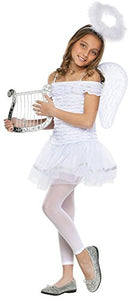# Little Angel Kids Costume

Vendor
Fun World
Regular price
\$53.24
Sale price
\$53.24
Quantity must be 1 or more

• S

• h

• e

• i

• s

• y

• o

• u

• r

• l

• i

• t

• t

• l

• e

• a

• n

• g

• e

• l

• a

• n

• d

• n

• o

• w

• s

• h

• e

• w

• a

• n

• t

• s

• t

• o

• d

• r

• e

• s

• s

• l

• i

• k

• e

• o

• n

• e

• !

• T

• h

• i

• s

• c

• o

• s

• t

• u

• m

• e

• i

• n

• c

• l

• u

• d

• e

• s

• d

• r

• e

• s

• s

• ,

• w

• i

• n

• g

• s

• a

• n

• d

• h

• a

• l

• o

• h

• e

• a

• d

• b

• a

• n

• d

• .

• D

• o

• e

• s

• n

• o

• t

• i

• n

• c

• l

• u

• d

• e

• n

• e

• c

• k

• l

• a

• c

• e

• ,

• t

• i

• g

• h

• t

• s

• ,

• h

• a

• r

• p

• ,

• o

• r

• s

• h

• o

• e

• s

• .

• Color : White

• Size : Small (4-6)

Includes: Dress, wings, headband. Not included: Harp, jewelry, tights, shoes.